# Rational numbers exercise 8.1 solutions class 7 NCERT

NCERT mathematics class 7 Rational Numbers Chapter 8 exercise 8.1 solutions are given.

You should study the lesson Rational Numbers very well.

You should also practice all example problems and solutions given in the textbook.

Observe the solutions and try them in your own method.

NCERT maths class 7th solutions

Rational Numbers

Exercise 8.1

Exercise 8.2

## Maths class 7 exercise 8.1 solutions NCERT Rational Numbers

Rational Numbers

chapter 8

Exercise 8.1

m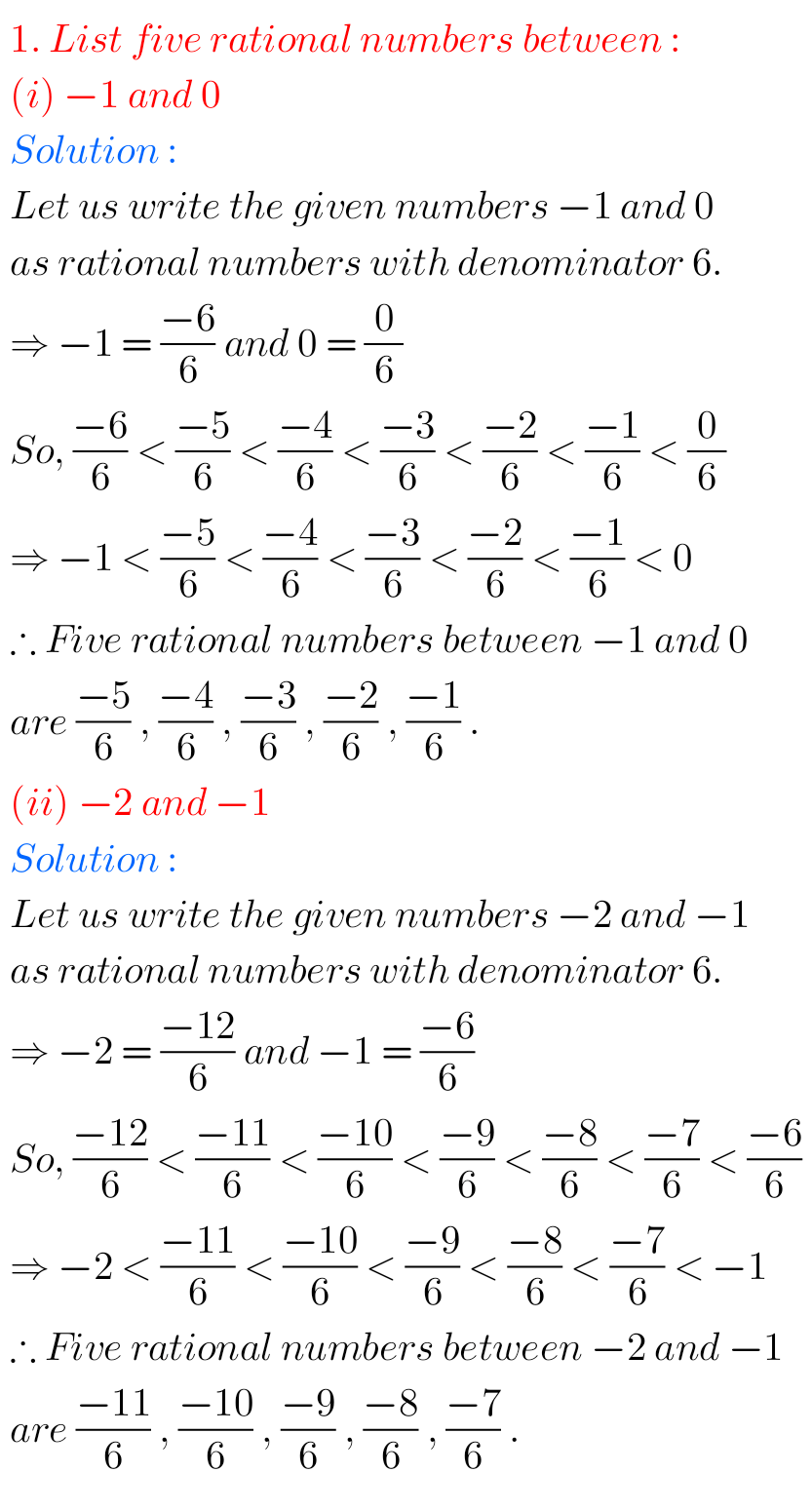mmm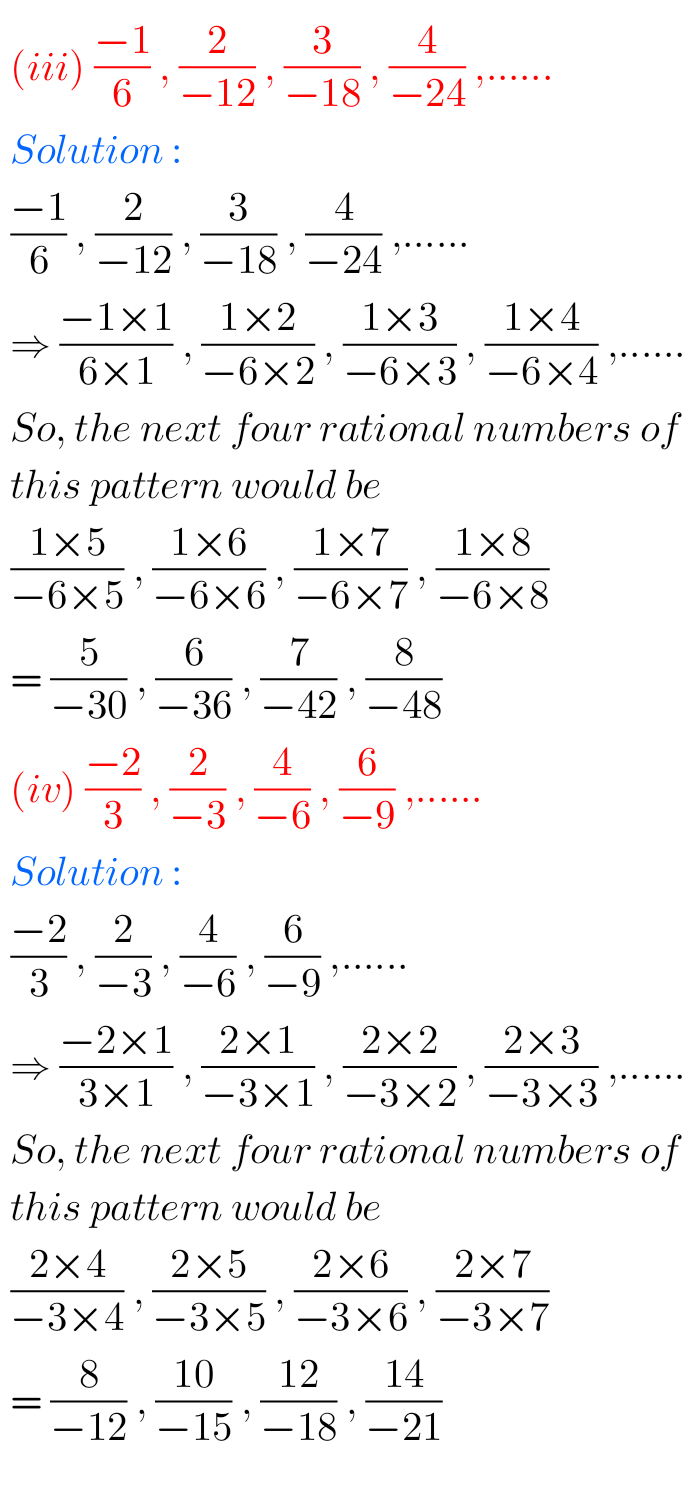mm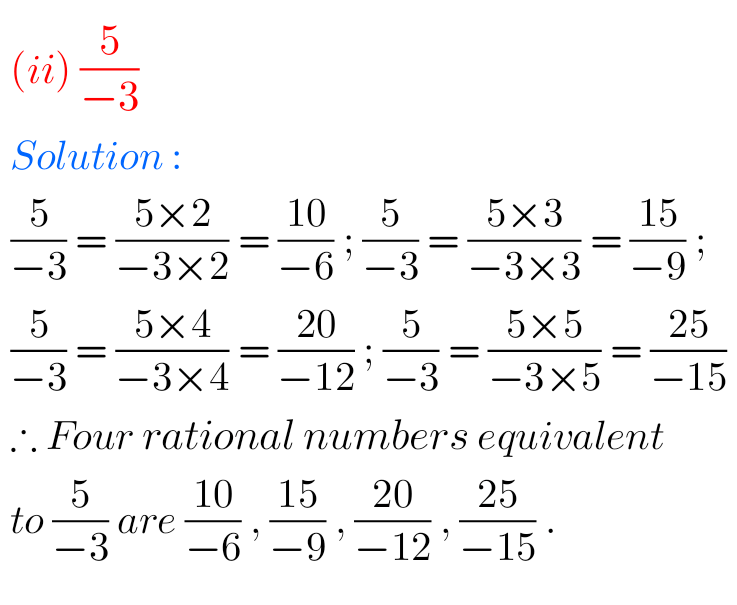m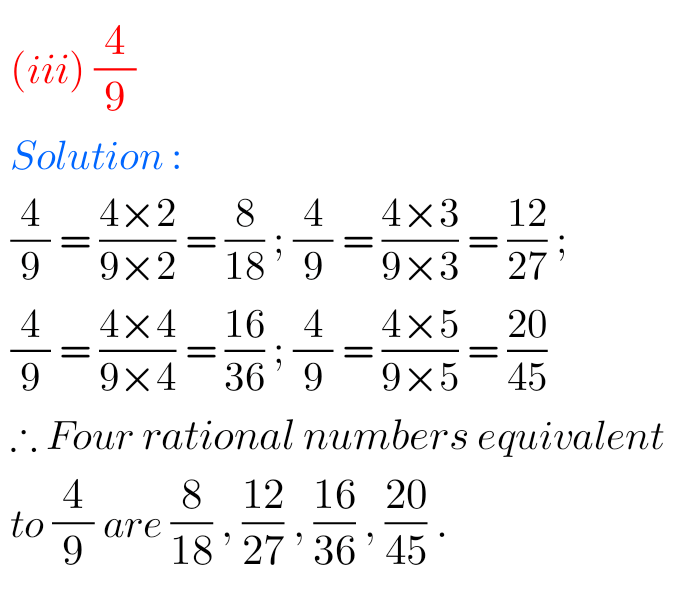mm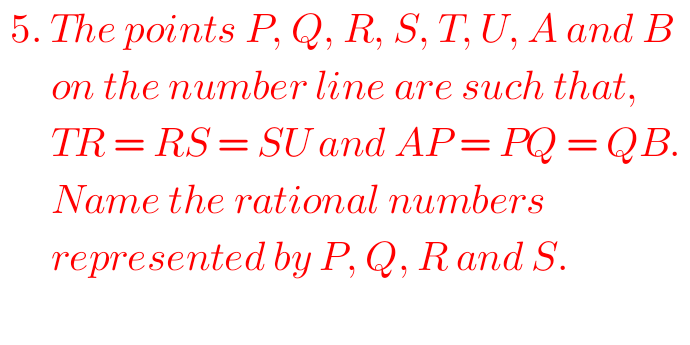mm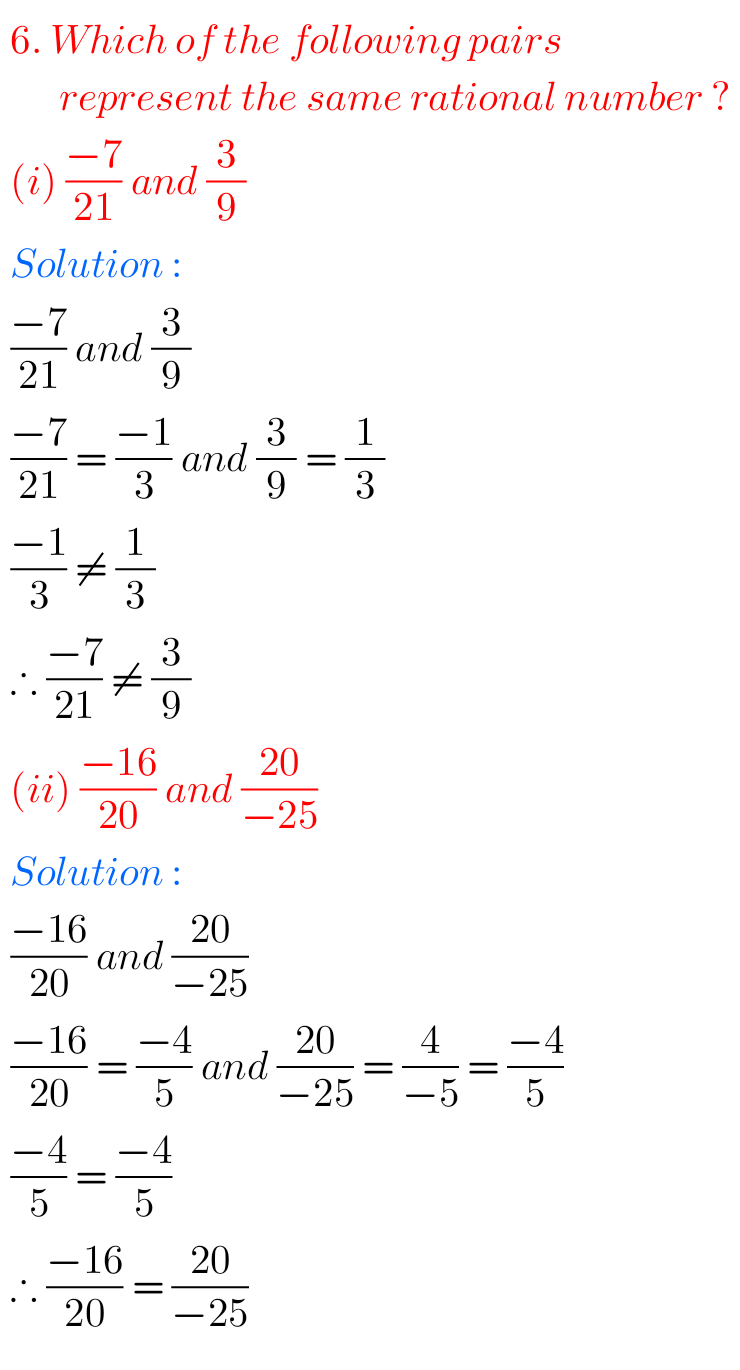mm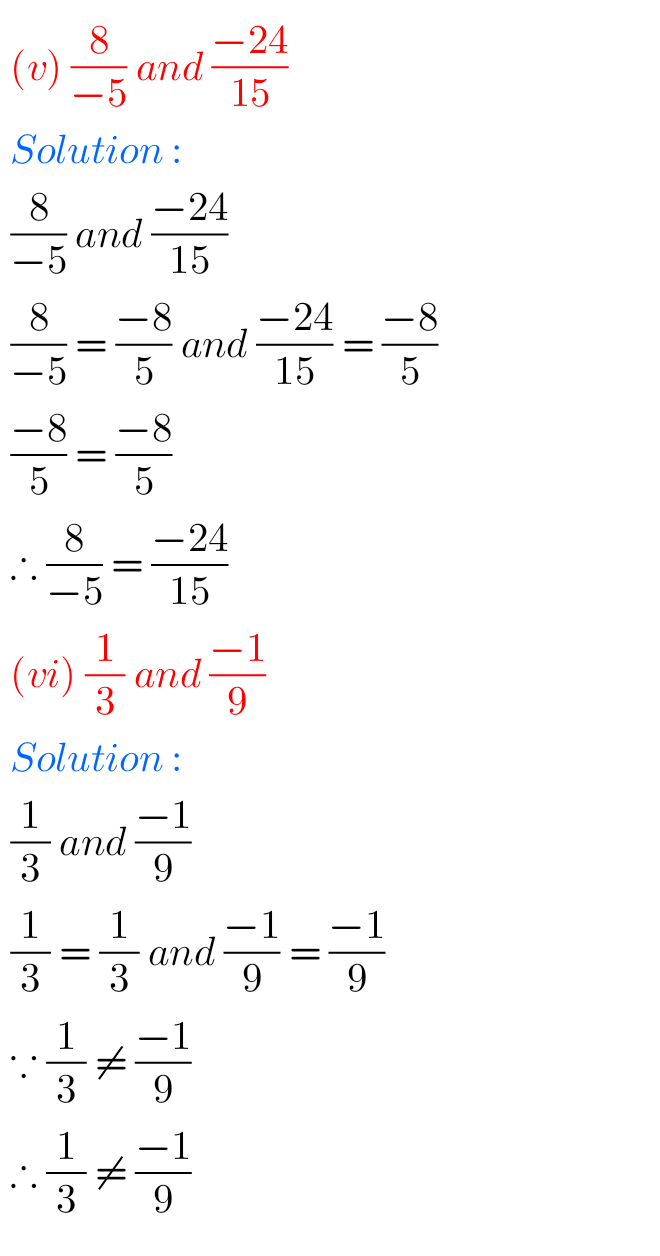m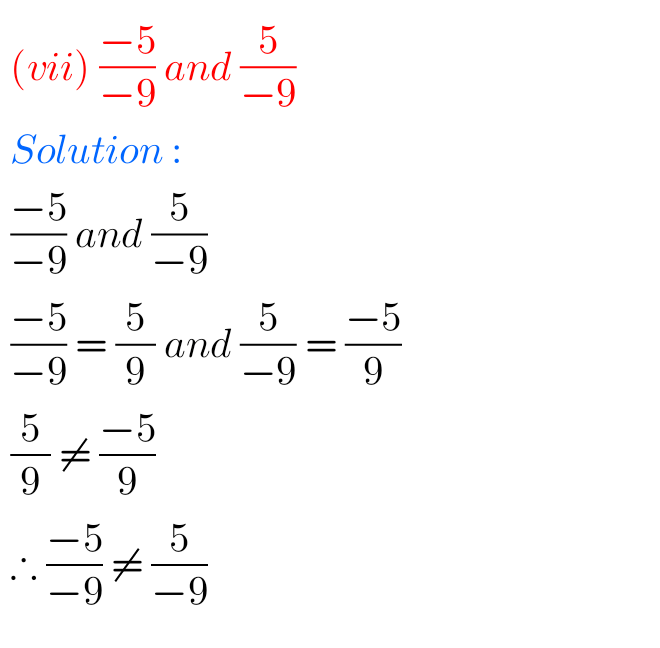m

### Solutions for Rational Numbers exercise 8.1 class 7 NCERT

m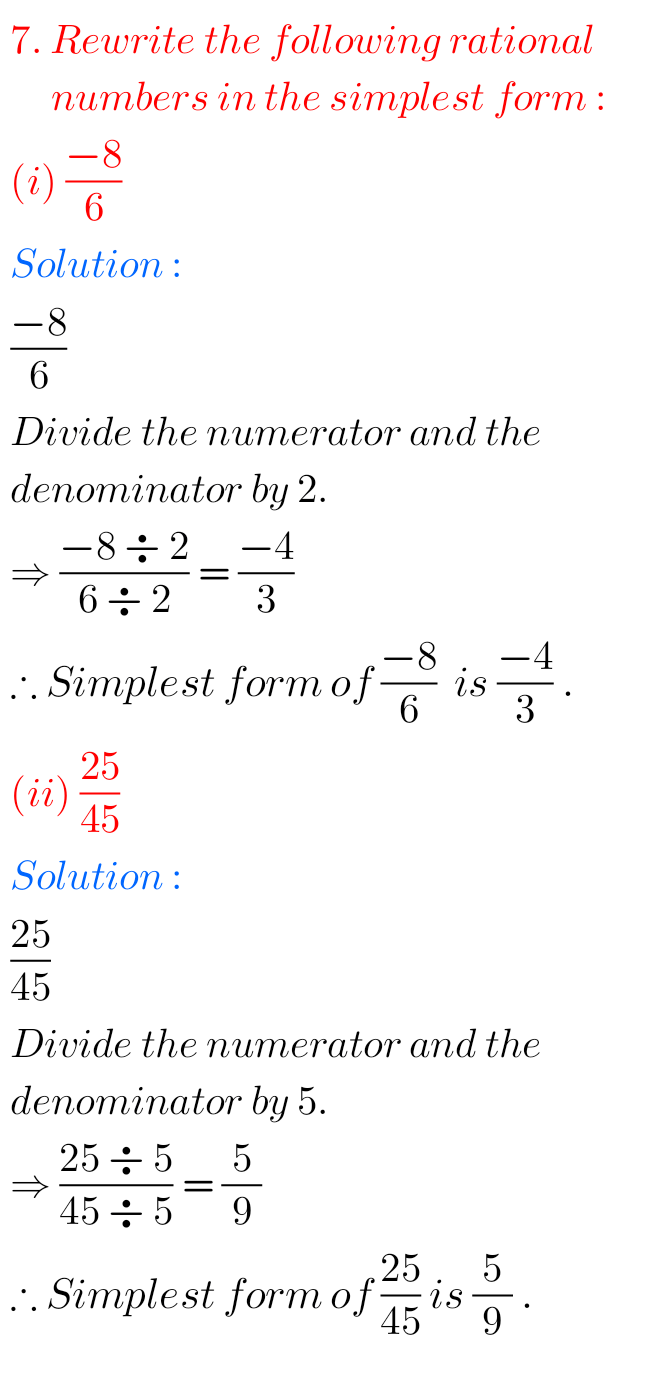mmm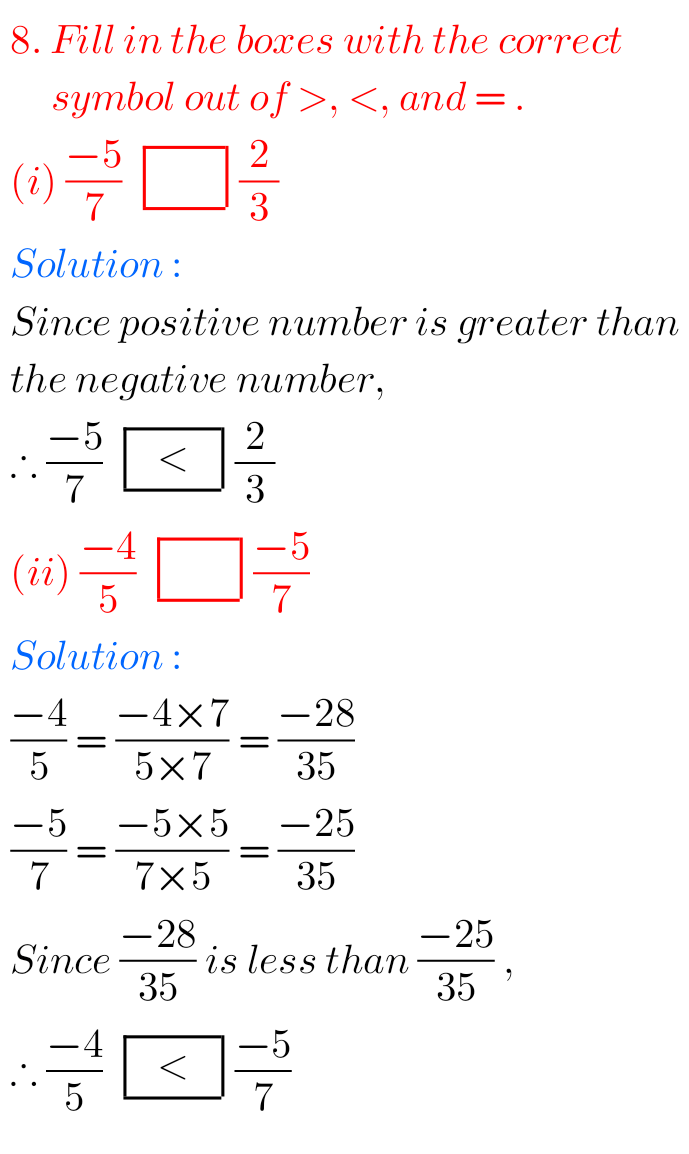m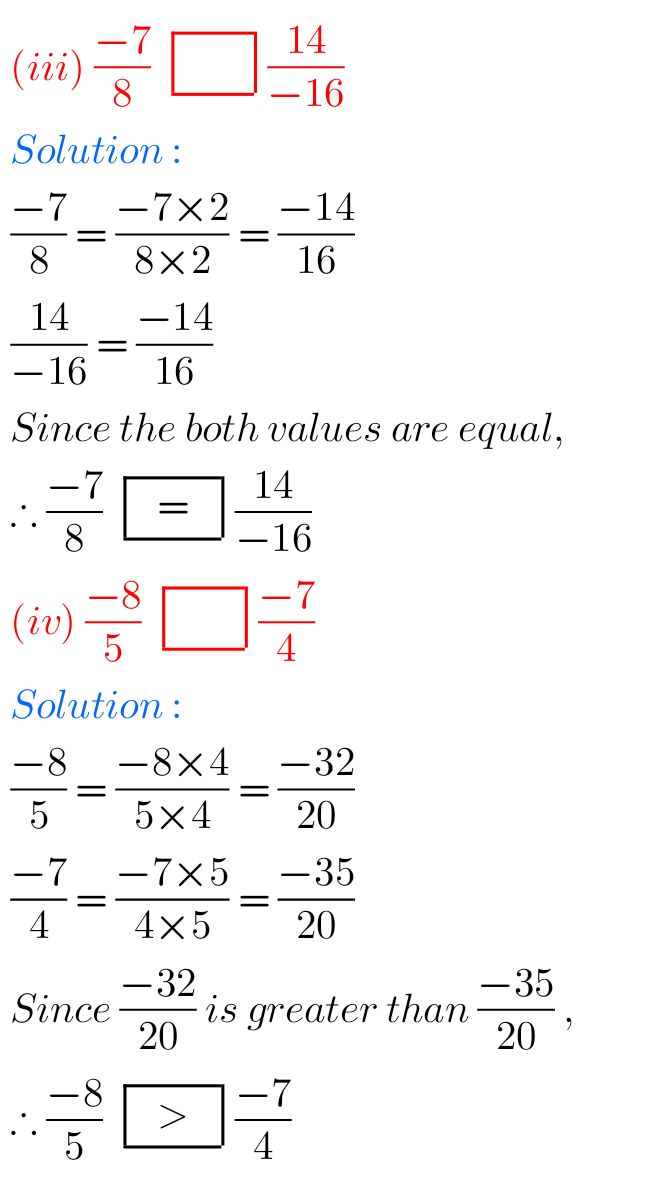mm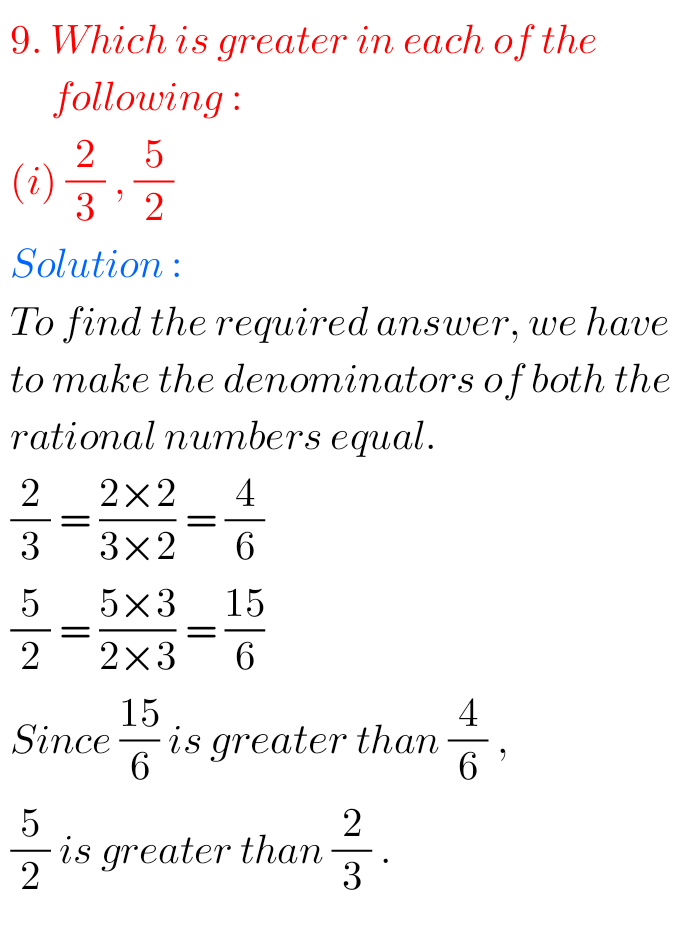m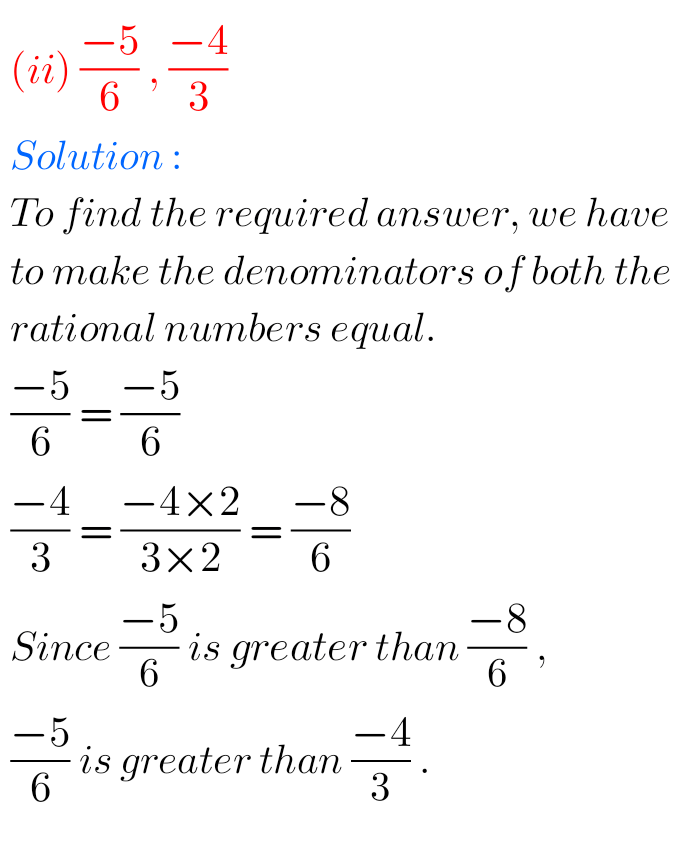m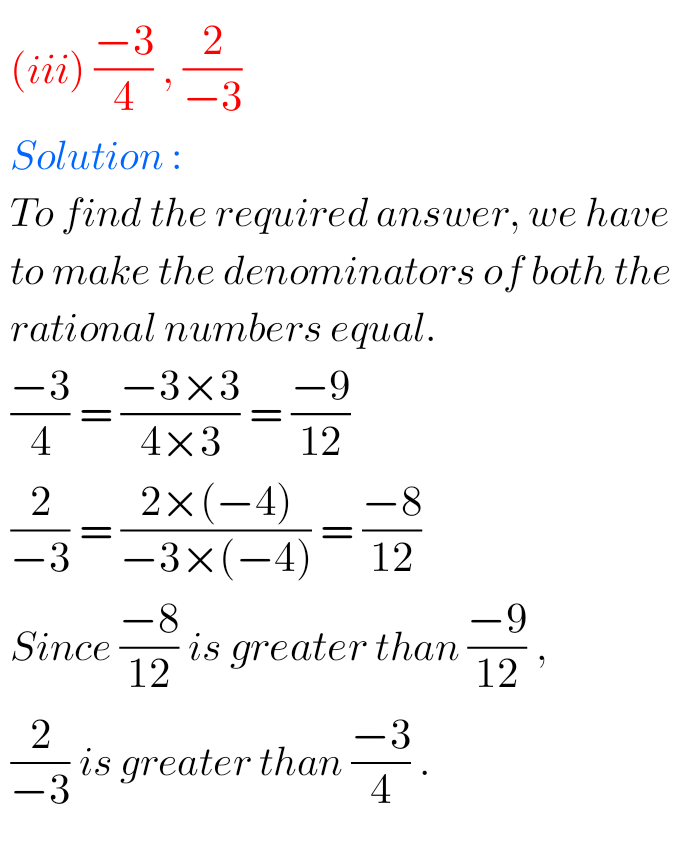mm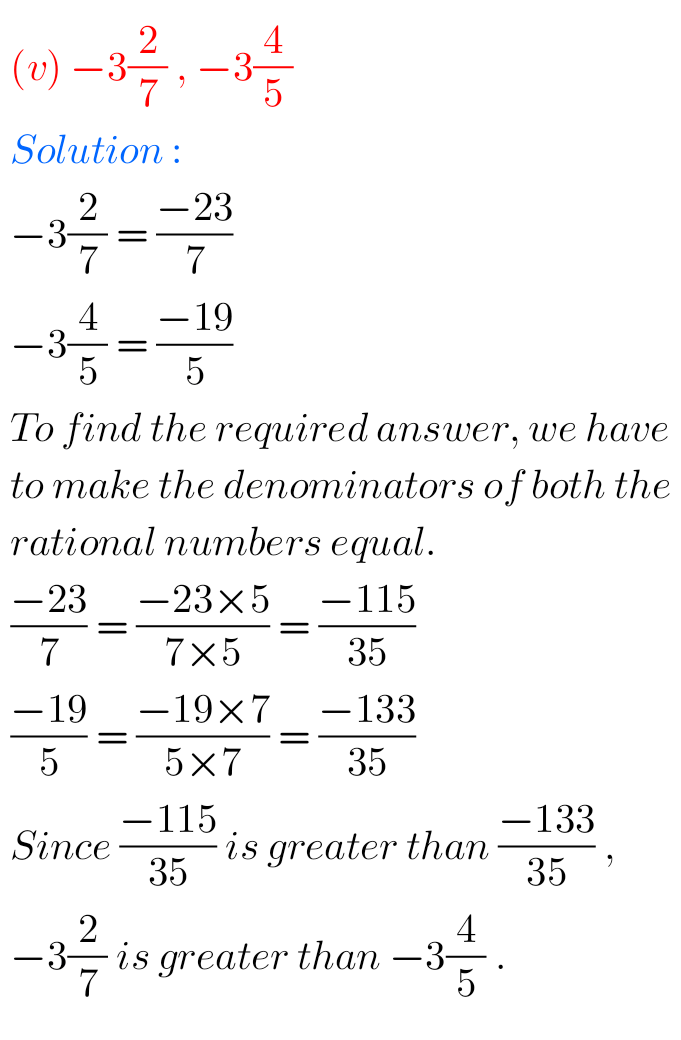m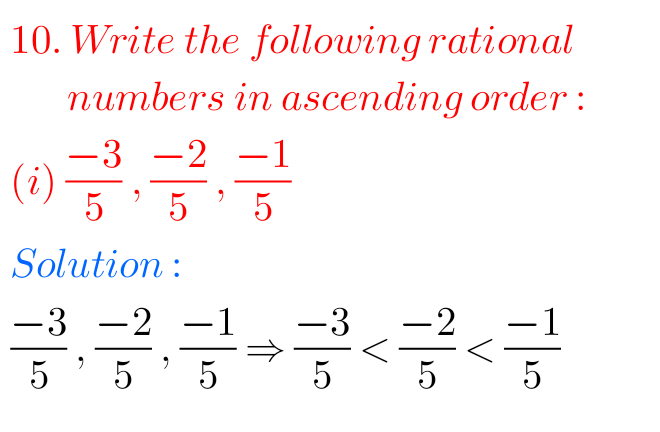mmNote: Observe the solutions and try them in your own method.

Ncert maths solutions for class 7th

NCERT maths solutions for class 6th

SSC maths solutions for class 10th

Inter maths solutions

NIOS Maths 311 book 2 solutions for some chapters